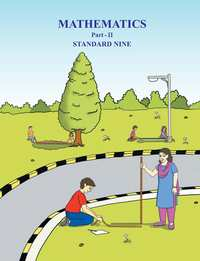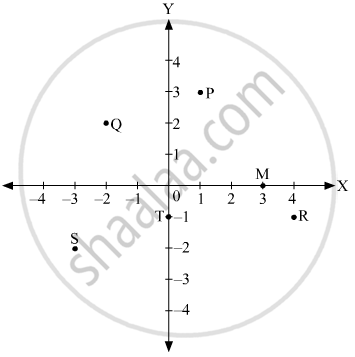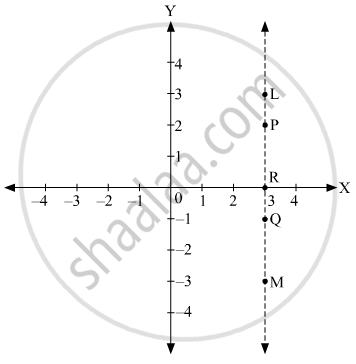Share

# Balbharati solutions for Class 9 chapter 7 - Co-ordinate Geometry [Latest edition]

#### Chapters## Chapter 7: Co-ordinate Geometry

Practice Set 7.1Practice Set 7.2Problem Set 7

#### Balbharati solutions for Class 9 Chapter 7 Exercise Practice Set 7.1 [Page 93]

Practice Set 7.1 | Q 1 | Page 93

State in which quadrant or on which axis do the following points lie.

1. A (-3 , 2)
2. B (-5 , -2)
3. C (3.5 , 1.5)
4. D (2 , 10)
5. E (37 , 35)
6. F (15 , -18)
7. G (3 , -7)
8. H (0 , -5)
9. M (12 , 0)
10. N (0 , 9)
11. P (0 , 2.5)
12. Q (-7 , -3)
Practice Set 7.1 | Q 2 | Page 93

In which quadrant are the following points ?

1.  whose both co-ordinates are positive.
2.   whose both co-ordinates are negative.
3.   whose x co-ordinate is positive, and the y co-ordinate is negative.
4.   whose x co-ordinate is negative and y co-ordinate is positive.
Practice Set 7.1 | Q 3 | Page 93

Draw the co-ordinate system on a plane and plot the following points.

L(-2 , 4) , M (5 , 6) , N (-3 , -4) , P (2 , -3) , Q (6 , -5) , S (7 , 0) , T(0 , -5)

#### Balbharati solutions for Class 9 Chapter 7 Exercise Practice Set 7.2 [Pages 97 - 98]

Practice Set 7.2 | Q 1 | Page 97

On a graph paper plot the points A (3,0), B(3,3), C(0,3). Join A, B and B, C. What is the figure formed?

Practice Set 7.2 | Q 2 | Page 97

Write the equation of the line parallel to the Y-axis at a distance of 7 units from it to its left.

Practice Set 7.2 | Q 3 | Page 97

Write the equation of the line parallel to the X-axis at a distance of 5 units from it and below the X-axis.

Practice Set 7.2 | Q 4 | Page 97

The point Q(- 3,-2) lies on a line parallel to the Y-axis. Write the equation of the line and draw its graph.

Practice Set 7.2 | Q 5 | Page 98

X-axis and line x = - 4 are parallel lines. What is the distance between them?

Practice Set 7.2 | Q 6.1 | Page 98

Which of the equation given below have graph parallel to the X-axis, and which ones have graph parallel to the Y-axis ?

x = 3

Practice Set 7.2 | Q 6.2 | Page 98

Which of the equation given below have graph parallel to the X-axis, and which ones have graph parallel to the Y-axis ?

y - 2 = 0

Practice Set 7.2 | Q 6.3 | Page 98

Which of the equation given below have graph parallel to the X-axis, and which ones have graph parallel to the Y-axis ?

x + 6 = 0

Practice Set 7.2 | Q 6.4 | Page 98

Which of the equation given below have graph parallel to the X-axis, and which ones have graph parallel to the Y-axis ?

y = - 5

Practice Set 7.2 | Q 7 | Page 98

On a graph paper, plot the points A(2, 3), B(6,-1) and C(0, 5). If those points are collinear then draw the line which includes them. Write the co-ordinates of the points at which the line intersects the X-axis and the Y-axis.

Practice Set 7.2 | Q 8 | Page 98
Draw the graphs of the following equations on the same system of co-ordinates. Write the co-ordinates of their points of intersection.
x + 4 = 0,      y - 1 = 0,      2x + 3 = 0,     3y - 15 = 0
Practice Set 7.2 | Q 9.1 | Page 98

Draw the graph of the equation given below

x + y = 2

Practice Set 7.2 | Q 9.2 | Page 98

Draw the graph of the equation given below

3x -  y = 0

Practice Set 7.2 | Q 9.3 | Page 98

Draw the graph of the equation given below

2x + y = 1

#### Balbharati solutions for Class 9 Chapter 7 Exercise Problem Set 7 [Pages 98 - 99]

Problem Set 7 | Q 1.1 | Page 98

Choose the correct alternative answer for the following quesiton.

What is the form of co-ordinates of a point on the X-axis ?

• ( b , b )

• ( o , b )

• ( a , o )

• ( a , a )

Problem Set 7 | Q 1.2 | Page 98

Choose the correct alternative answer for the following quesiton.

Any point on the line y = x is of the form .....

• ( a , a )

• ( o , a )

• ( a , o )

• ( a , - a )

Problem Set 7 | Q 1.3 | Page 98

Choose the correct alternative answer for the following quesiton.

What is the equation of the X-axis ?

• x = 0

• y = 0

• x + y = 0

• x = y

Problem Set 7 | Q 1.4 | Page 98

Choose the correct alternative answer for the following quesiton.

In which quadrant does the point ( - 4, - 3) lie ?

• First

• Second

• Third

• Fourth

Problem Set 7 | Q 1.5 | Page 98

Choose the correct alternative answer for the following quesiton.

What is the nature of the line which includes the points
( -5,5), (6,5), (- 3,5), (0,5) ?

• Passes through the origin

• Parallel to Y-axis

• Parallel to X-axis

• None of these

Problem Set 7 | Q 1.6 | Page 98

Choose the correct alternative answer for the following quesiton.

Which of the points P ( -1,1), Q (3,- 4), R(1 , -1), S ( -2, - 3), T (- 4,4) lie in the fourth quadrant ?

• P and T

• Q and R

• only S

• P and R

Problem Set 7 | Q 2 | Page 99

Some points are shown in the given figure, With the help of it answer the following questions :1. Write the co-ordinates of the points Q and R.
2. Write the co-ordinates of the points T and M.
3. Which point lies in the third quadrant ?
4. Which are the points whose x and y co-ordinates are equal ?

Problem Set 7 | Q 3.1 | Page 99

Without plotting the point on a graph, state in which quadrant or on which axis do the following point lie.

(5 , -3)

Problem Set 7 | Q 3.2 | Page 99

Without plotting the point on a graph, state in which quadrant or on which axis do the following point lie.

(-7 , -12)

Problem Set 7 | Q 3.3 | Page 99

Without plotting the point on a graph, state in which quadrant or on which axis do the following point lie.

(-23 , 4)

Problem Set 7 | Q 3.4 | Page 99

Without plotting the point on a graph, state in which quadrant or on which axis do the following point lie.

(-9 , 5)

Problem Set 7 | Q 3.5 | Page 99

Without plotting the point on a graph, state in which quadrant or on which axis do the following point lie.

(0 , -3)

Problem Set 7 | Q 3.6 | Page 99

Without plotting the point on a graph, state in which quadrant or on which axis do the following point lie.

(-6 , 0)

Problem Set 7 | Q 4 | Page 99
Plot the following points on the one and the same co-ordinate system.
A(1, 3),  B(- 3, - 1), C(1, - 4),   D(- 2, 3), E(0, - 8), F(1, 0)
Problem Set 7 | Q 5 | Page 99

In the graph alongside, line LM is parallel to the Y-axis.1.  What is the distance of line LM from the Y-axis ?

2.  Write the co-ordinates of the points P, Q and R.

3.  What is the difference between the x co-ordinates of the points L and M?
Problem Set 7 | Q 6 | Page 99

How many lines are there which are parallel to X-axis and having a distance 5 units?

Problem Set 7 | Q 7 | Page 99

If ‘a’ is a real number, what is the distance between the Y-axis and the line x = a ?

## Chapter 7: Co-ordinate Geometry

Practice Set 7.1Practice Set 7.2Problem Set 7## Balbharati solutions for Class 9 Geometry chapter 7 - Co-ordinate Geometry

Balbharati solutions for Class 9 chapter 7 (Co-ordinate Geometry) include all questions with solution and detail explanation. This will clear students doubts about any question and improve application skills while preparing for board exams. The detailed, step-by-step solutions will help you understand the concepts better and clear your confusions, if any. Shaalaa.com has the Maharashtra State Board Balbharati Class 9 Mathematics 2 Geometry solutions in a manner that help students grasp basic concepts better and faster.

Further, we at Shaalaa.com provide such solutions so that students can prepare for written exams. Balbharati textbook solutions can be a core help for self-study and acts as a perfect self-help guidance for students.

Concepts covered in Class 9 Geometry chapter 7 Co-ordinate Geometry are Introduction of Coordinate Geometry, Cartesian System, To Plot the Points of Given Co-ordinates, Equations of Lines Parallel to the X-axis and Y-axis, Graph of a Linear Equations.

Using Balbharati Class 9 solutions Co-ordinate Geometry exercise by students are an easy way to prepare for the exams, as they involve solutions arranged chapter-wise also page wise. The questions involved in Balbharati Solutions are important questions that can be asked in the final exam. Maximum students of Maharashtra State Board Class 9 prefer Balbharati Textbook Solutions to score more in exam.

Get the free view of chapter 7 Co-ordinate Geometry Class 9 extra questions for and can use Shaalaa.com to keep it handy for your exam preparation

S